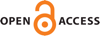Download this articleFor screen For printingRecent IssuesThe Journal About the Journal Editorial Board Editors’ Interests Subscriptions Submission Guidelines Submission Form Policies for Authors Ethics Statement ISSN: 1948-206X (e-only) ISSN: 2157-5045 (print) Author Index To Appear Other MSP JournalsRiesz transform and vertical oscillation in the Heisenberg group

### Katrin Fässler and Tuomas Orponen

Vol. 16 (2023), No. 2, 309–340##### Abstract

We study the ${L}^{2}$-boundedness of the $3$-dimensional (Heisenberg) Riesz transform on intrinsic Lipschitz graphs in the first Heisenberg group $ℍ$. Inspired by the notion of vertical perimeter, recently defined and studied by Lafforgue, Naor, and Young, we first introduce new scale and translation invariant coefficients ${\mathrm{osc}}_{\mathrm{\Omega }}\left(B\left(q,r\right)\right)$. These coefficients quantify the vertical oscillation of a domain $\mathrm{\Omega }\subset ℍ$ around a point $q\in \partial \mathrm{\Omega }$, at scale $r>0$. We then proceed to show that if $\mathrm{\Omega }$ is a domain bounded by an intrinsic Lipschitz graph $\Gamma$, and

 ${\int }_{0}^{\infty }{\mathrm{osc}}_{\mathrm{\Omega }}\left(B\left(q,r\right)\right)\frac{dr}{r}\le C<\infty ,\phantom{\rule{1em}{0ex}}q\in \Gamma ,$

then the Riesz transform is ${L}^{2}$-bounded on $\Gamma$. As an application, we deduce the boundedness of the Riesz transform whenever the intrinsic Lipschitz parametrisation of $\Gamma$ is an $𝜖$ better than $\frac{1}{2}$-Hölder continuous in the vertical direction.

We also study the connections between the vertical oscillation coefficients, the vertical perimeter, and the natural Heisenberg analogues of the $\beta$-numbers of Jones, David, and Semmes. Notably, we show that the ${L}^{p}$-vertical perimeter of an intrinsic Lipschitz domain $\mathrm{\Omega }$ is controlled from above by the $p$-th powers of the ${L}^{1}$-based $\beta$-numbers of $\partial \mathrm{\Omega }$.

##### Keywords
Singular integrals, Riesz transform, intrinsic Lipschitz graphs, Heisenberg group
##### Mathematical Subject Classification 2010
Primary: 42B20
Secondary: 28A78, 31C05, 32U30, 35R03
##### Milestones